# SCERT Maharashtra Question Bank solutions for 10th Standard SSC Science and Technology 1 Maharashtra State Board 2021 chapter 7 - Lenses [Latest edition]

#### Chapters## Chapter 7: Lenses

Choose the correct option.Find the correlation.Find odd one out.Write the nameRight or Wrong sentenceWrite an ExplanationWrite scientific reasonsDistinguish betweenAnswer the FollowingAnswer the following questions
Choose the correct option.

### SCERT Maharashtra Question Bank solutions for 10th Standard SSC Science and Technology 1 Maharashtra State Board 2021 Chapter 7 Lenses Choose the correct option.

#### Choose the correct option.

Choose the correct option. | Q 1

A ray of light gets refracted _______ while entering the lens.

• once

• twice

• thrice

• doesn’t happen

Choose the correct option. | Q 2

The point inside the lens on the principal axis through which light rays pass without changing their path is called _______.

• centre of curvature

• optical centre

• principal focus

• axiom point

Choose the correct option. | Q 3

Virtual image is formed by a convex lens if an object is placed _______.

• at infinity

• at 2F1

• at focus F1

• between F1 and O

Choose the correct option. | Q 4

In the convex lens if an object is placed at 2 F1, the image is formed at ______.

• F1

• 2 F2

• beyond 2 F1

• On the same side of the lens as the object

Choose the correct option. | Q 5

All distances parallel to the principal axis are measured from the _______.

• optical centre

• centre of curvature

• principal focus

• infinity

Choose the correct option. | Q 6

A small hole of changing diameter at the centre of Iris is called _______.

• optic nerves

• cornea

• optic disc

• pupil

Choose the correct option. | Q 7

For a normal human eye the near point is at _______.

• 2.1 cm

• 2.5 cm

• 25 cm

• 5 cm

Choose the correct option. | Q 8

The image formed by _______ lens is always virtual and small.

• plane convex

• biconvex

• biconcave

• bifocal

Choose the correct option. | Q 9

In a relaxed state, the focal length of healthy eyes is _______.

• 2 cm

• 2.5 cm

• 25 cm

• 5 cm

Choose the correct option. | Q 10

For a specific glass lens f = 0.5 m. This is the only information given to the student. Which type of lens is given to him and what is its power?

• power 2 D; convex lens

• power 1 D; concave lens

• power - 0.5 D; concave lens

• power - 0.25 D; convex lens

Choose the correct option. | Q 11

In Myopia the human eye _______.

• cannot see nearby objects distinctly

• cannot see distant objects clearly

• cannot see nearby as well as distant objects clearly

• can see nearby as well as distant objects clearly

Choose the correct option. | Q 12

Due to elongation of _______ and increase in curvature of the eye lens, a person cannot see distant objects clearly.

• eyeball

• pupil

• eyelid

• cornea

Choose the correct option. | Q 13

In hypermetropia human eye _______.

• can see distant objects clearly

• can see nearby objects distinctly

• cannot see nearby as well as distant objects clearly

• can see nearby as well as distant objects clearly

Choose the correct option. | Q 14

Bifocal lens is required to correct _______ defect.

• myopia

• hypermetropia

• presbyopia

• none of these

Choose the correct option. | Q 15

_______ times larger images can be obtained by using a simple microscope.

• 5

• 10

• 20

• 60

Choose the correct option. | Q 16

_______ is a combination of two convex lenses with small focal length.

• simple microscope

• compound microscope

• telescope

• none of these

Find the correlation.

### SCERT Maharashtra Question Bank solutions for 10th Standard SSC Science and Technology 1 Maharashtra State Board 2021 Chapter 7 Lenses Find the correlation.

#### Find the correlation.

Find the correlation. | Q 1

Convex lens : converging : : concave lens : _______

Find the correlation. | Q 2

Nearsightedness: elongated eyeball : : farsightedness: _______

Find the correlation. | Q 3

Object at 2F1 of a convex lens : Image at 2F2 : : Object at F1 : _______

Find the correlation. | Q 4

Nearsightedness : concave lens : : farsightedness : _______

Find the correlation. | Q 5

Simple microscope : Number of convex lens one : : compound microscope : _______

Find the correlation. | Q 6

Focal length : metre : : power of lens : _______

Find the correlation. | Q 7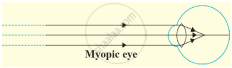: Concave lens : :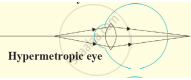: ______

Find the correlation. | Q 8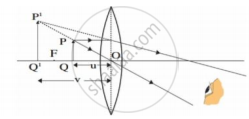: Object near the lens : : ______ :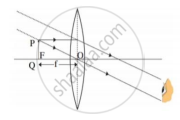Find odd one out.

### SCERT Maharashtra Question Bank solutions for 10th Standard SSC Science and Technology 1 Maharashtra State Board 2021 Chapter 7 Lenses Find odd one out.

Find odd one out. | Q 1

Find the odd one out and give its explanation.

• Focal length

• Image distance

• Size of image

Find odd one out. | Q 2

Find the odd one out and give its explanation.

• Simple microscope

• Compound microscope

• Telescope

• Prism

Find odd one out. | Q 3

Find the odd one out and give its explanation.

• Eye lens

• Retina

• Cerebellum

• Cornea

Find odd one out. | Q 4

Find the odd one out and give its explanation.

• Object distance

• Image distance

• Focal length

• Principal axis

Find odd one out. | Q 5

Find the odd one out and give its explanation.

• Eye piece

• Magnifier

• Kaleidoscope

• Telescope

Write the name

### SCERT Maharashtra Question Bank solutions for 10th Standard SSC Science and Technology 1 Maharashtra State Board 2021 Chapter 7 Lenses Write the name

Write the name | Q 1

Write the name.

The distance between focus and optical centre.

Write the name | Q 2

Write the name.

The part of human eye that transmits electrical signals to the brain.

Write the name | Q 3

Write the name.

The lens used in simple microscope.

Write the name | Q 4

Write the name.

The ability of lens to adjust the focal length as per need is

Write the name | Q 5

Write the name.

The defect of eye occurring due to ageing

Write the name | Q 6

Write the name.

The fleshy screen behind cornea.

Write the name | Q 7

Write the name.

The screen with light sensitive cells in human eye.

Write the name | Q 8

Write the name.

The sensation on the retina persists for a while is

Write the name | Q 9

Write the name.

The persons which are unable to distinguish between different colours

Write the name | Q 10

Write the name.

The imaginary line passing through two optical centres of lens

Right or Wrong sentence

### SCERT Maharashtra Question Bank solutions for 10th Standard SSC Science and Technology 1 Maharashtra State Board 2021 Chapter 7 Lenses Right or Wrong sentence

Right or Wrong sentence | Q 1

Convex magnifying glass is called divergent magnifying glass and concave magnifying glass is called converging magnifying glass.

• Right

• Wrong

Right or Wrong sentence | Q 2

The image of the object in the human eye is formed on the cross screen.

• Right

• Wrong

Right or Wrong sentence | Q 3

Hypermetropia can be corrected using concave lens of proper focal length.

• Right

• Wrong

Right or Wrong sentence | Q 4

When the incident ray is parallel to the principal axis, the refracted ray passes through the principal focus.

• Right

• Wrong

Right or Wrong sentence | Q 5

The image of an object at an infinite distance is obtained in a real and erect form through a convex magnifying glass.

• Right

• Wrong

Right or Wrong sentence | Q 6

The power of the magnifying glass depends on the distance of the magnifying glass from object.

• True

• Wrong

Right or Wrong sentence | Q 7

The lens of the eye is flattened when looking at nearby objects.

• Right

• Wrong

Right or Wrong sentence | Q 8

For a healthy human eye, the distant point is infinite distance.

• Right

• Wrong

Right or Wrong sentence | Q 9

Vision defect that increases distance between the lens of the eye and retina of the eye is termed as myopia.

• Right

• Wrong

Right or Wrong sentence | Q 10

The virtual image of object seen by the eye depends on the angle subtended by the object with the eye.

• Right

• Wrong

Write an Explanation

### SCERT Maharashtra Question Bank solutions for 10th Standard SSC Science and Technology 1 Maharashtra State Board 2021 Chapter 7 Lenses Write an Explanation

Write an Explanation | Q 1

Write an Explanation.

Centre of curvature of the lens

Write an Explanation | Q 2

Write an Explanation.

The optical centre of the lens

Write an Explanation | Q 3

Write an Explanation.

Principal focus

Write an Explanation | Q 4

Write an Explanation.

Focal length

Write an Explanation | Q 5

Write an Explanation.

Principal axis

Write an Explanation | Q 6

Write an Explanation.

Minimum distance of distinct vision

Write an Explanation | Q 7

Write an Explanation.

Farthest distance of distinct vision

Write an Explanation | Q 8

Write an Explanation.

Magnification

Write an Explanation | Q 9

Write an Explanation.

Power of accommodation

Write an Explanation | Q 10

Write an Explanation.

Persistence of vision

Write scientific reasons

### SCERT Maharashtra Question Bank solutions for 10th Standard SSC Science and Technology 1 Maharashtra State Board 2021 Chapter 7 Lenses Write scientific reasons

Write scientific reasons | Q 1

Write scientific reason.

A convex lens is called a converging lens.

Write scientific reasons | Q 2

Write scientific reason.

Nearsightedness, this defect can be corrected by using spectacles with concave lens.

Write scientific reasons | Q 3

Write scientific reason.

Farsightedness, this defect can be corrected by using convex lens.

Write scientific reasons | Q 4

Write scientific reason.

Write scientific reasons | Q 5

Write scientific reason.

Presbyopia effect is more common in people over 40 years of age.

Write scientific reasons | Q 6

Give scientific reason.

Simple microscope is used for watch repairs.

Write scientific reasons | Q 7

Give scientific reason:
One can sense colours only in bright light.

Write scientific reasons | Q 8

Write scientific reason.

The movie cannot be enjoyed if seat of a viewer is too close to the screen in the cinema.

Write scientific reasons | Q 9

Give scientific reason:

We cannot clearly see an object kept at a distance less than 25 cm from the eye.

Distinguish between

### SCERT Maharashtra Question Bank solutions for 10th Standard SSC Science and Technology 1 Maharashtra State Board 2021 Chapter 7 Lenses Distinguish between

Distinguish between | Q 1

Distinguish between:

Concave lens and Convex lens.

Distinguish between | Q 2

Distinguish between:

Farsightedness and Nearsightedness.

Distinguish between | Q 3

Distinguish between:

Myopia - Presbyopia

### SCERT Maharashtra Question Bank solutions for 10th Standard SSC Science and Technology 1 Maharashtra State Board 2021 Chapter 7 Lenses Answer the Following

Answer the Following | Q 1

Find the power of a convex lens of focal length of + 25 cm.

Answer the Following | Q 2

If focal length of a convex lens is 20 cm at what is the power of the lens?

Answer the Following | Q 3

If each two concave lenses of focal length 30 cm are kept in contact with each other what will be the power of combination?

Answer the Following | Q 4

An object is placed at a distance of 10 cm from a convex lens of focal length 12 cm, find out at what distance image will be formed from the lens and what is the nature of image.

Answer the Following | Q 5

5 cm high object is placed at a distance of 10 cm from a converging lens of focal length of 20 cm. Determine the position, size and type of the image.

Answer the Following | Q 6

An object is placed vertically at a distance of 20 cm from a convex lens. If the height of the object is 5 cm and the focal length of the lens is 10 cm, what will be the position, size and nature of the image? How much bigger as compared to the object?

Answer the Following | Q 7

Two convex lenses of focal length 30 cm and 10 cm each are kept in contact with each other. Find the power of their combination.

Answer the Following | Q 8.1

The following figure show the change in the shape of the lens while seeing distant and nearby objects. Complete the figures by correctly labelling the diagram.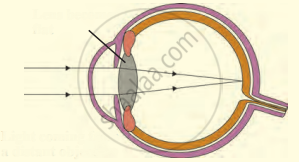Answer the Following | Q 8.2

The following figure show the change in the shape of the lens while seeing distant and nearby objects. Complete the figures by correctly labelling the diagram.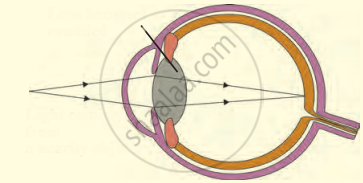Answer the Following | Q 9

Write the function of the human eye and label parts of the figure given below.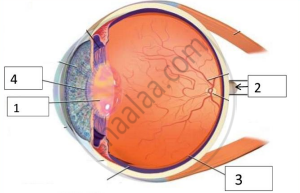Answer the Following | Q 10

Observe the given below the figure, correct it and explain and write about the concept depicted in this figure.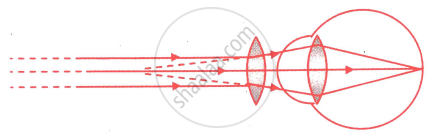Answer the Following | Q 11

Given below is a diagram showing a defect of vision. Name the defect of vision and draw an accurately labelled diagram to correct this defect.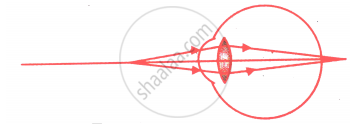Answer the Following | Q 12

Write law in a given figure.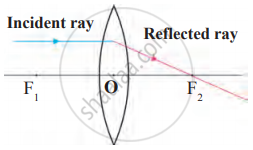Answer the Following | Q 12

Write law in a given figure.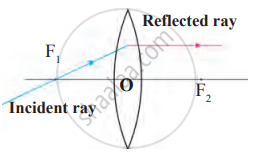Answer the Following | Q 12

Write law in a given figure.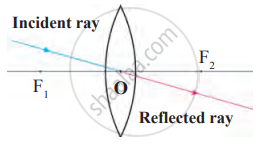Answer the Following | Q 13

Observe the given figure and answer the following questions.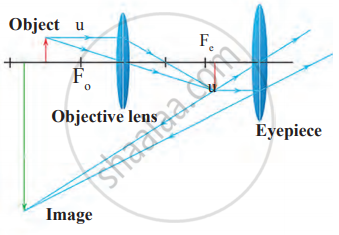1. Where is the above type of lens construction used?
2. What type of image is formed by an objective lens?
3. What happens instead of placing at Fo if the object is placed in between O and Fo?
Answer the Following | Q 14

Identify and explain concepts given in this diagram.Answer the Following | Q 15

Complete the paragraph by choosing the right options given below.

(minimum, near point, 25 cm, farthest, farthest distance, far point)

The _______ distance of an object from a normal eye, at which it is clearly visible without stress on the eye, is called the minimum distance of distinct vision. The position of the object at this distance is called the _______ of the eye, for a normal human eye, the near point is at _______. The _______ distance of an object from a human eye, at which it is clearly visible without stress on the eye is called _______ of distinct vision. The position of the object at this distance is called the _______ of the eye.

Answer the Following | Q 16

Choose the correct option from the bracket and complete the stanza.

(colour blind, actual, conical, light-sensitive, rodlike, colours)

The retina in our eyes is made up of many _______ cells. These cells are shaped like a rod and like a cone. The _______ cells respond to the intensity of light and give information about the brightness or dimness of the object to the brain. The _______ cells respond to the colour and give information about the colour of the object to the brain. Brain processes all the information received and we see the _______ image of the object. Rod like cells respond to the faint light also but ______ cells do not. Some people lack conical cells responding to certain colours. These persons cannot recognize those colours or cannot distinguish between different ______. These persons are said to be ______.

### SCERT Maharashtra Question Bank solutions for 10th Standard SSC Science and Technology 1 Maharashtra State Board 2021 Chapter 7 Lenses Answer the following questions

Answer the following questions | Q 1.1

Explain with a ray diagram the position, size, and nature of the various image formed by convex lenses.

An object is at infinity.

Answer the following questions | Q 1.2

Explain with a ray diagram the position, size, and nature of the various images formed by the convex lens.

An object beyond 2F1.

Answer the following questions | Q 1.3

Explain with a ray diagram the position, size, and nature of the various images formed by a convex lens.

An object at 2F1.

Answer the following questions | Q 1.4

Explain with a ray diagram the position, size, and nature of the various images formed by a convex lens.

An object is in between F1 and 2F1

Answer the following questions | Q 1.5

Explain with a ray diagram the position, size, and nature of the various images formed by a convex lens.

Object at focus F1

Answer the following questions | Q 1.6

Explain with a ray diagram the position, size, and nature of the various images formed by a convex lens.

Object between F1 and O

## Chapter 7: Lenses

Choose the correct option.Find the correlation.Find odd one out.Write the nameRight or Wrong sentenceWrite an ExplanationWrite scientific reasonsDistinguish betweenAnswer the FollowingAnswer the following questions## SCERT Maharashtra Question Bank solutions for 10th Standard SSC Science and Technology 1 Maharashtra State Board 2021 chapter 7 - Lenses

SCERT Maharashtra Question Bank solutions for 10th Standard SSC Science and Technology 1 Maharashtra State Board 2021 chapter 7 (Lenses) include all questions with solution and detail explanation. This will clear students doubts about any question and improve application skills while preparing for board exams. The detailed, step-by-step solutions will help you understand the concepts better and clear your confusions, if any. Shaalaa.com has the Maharashtra State Board 10th Standard SSC Science and Technology 1 Maharashtra State Board 2021 solutions in a manner that help students grasp basic concepts better and faster.

Further, we at Shaalaa.com provide such solutions so that students can prepare for written exams. SCERT Maharashtra Question Bank textbook solutions can be a core help for self-study and acts as a perfect self-help guidance for students.

Concepts covered in 10th Standard SSC Science and Technology 1 Maharashtra State Board 2021 chapter 7 Lenses are Power of a Lens, Eye Defect and its correction: Hypermetropia or far-sightedness, Eye Defect and Its Correction: Presbyopia, Convex Lens, Concave Lens, Images Formed by Sperical Lenses, Lens, Spherical Lens, Guideline for Image Formation Due to Refraction Through a Convex and Concave Lens, Images Formed Due to Refraction Through a Concave Lens, Images Formed Due to Refraction Through a Convex Lens, Sign Convention for Spherical Lenses, Lens Formula, Magnification Due to Spherical Lenses, Combination of Lenses, Human Eye: Structure of the Eye, Working of the Human Eye, Eye Defect and Its Correction: Myopia Or Near-sightedness.

Using SCERT Maharashtra Question Bank 10th Standard [इयत्ता १० वी] solutions Lenses exercise by students are an easy way to prepare for the exams, as they involve solutions arranged chapter-wise also page wise. The questions involved in SCERT Maharashtra Question Bank Solutions are important questions that can be asked in the final exam. Maximum students of Maharashtra State Board 10th Standard [इयत्ता १० वी] prefer SCERT Maharashtra Question Bank Textbook Solutions to score more in exam.

Get the free view of chapter 7 Lenses 10th Standard [इयत्ता १० वी] extra questions for 10th Standard SSC Science and Technology 1 Maharashtra State Board 2021 and can use Shaalaa.com to keep it handy for your exam preparation